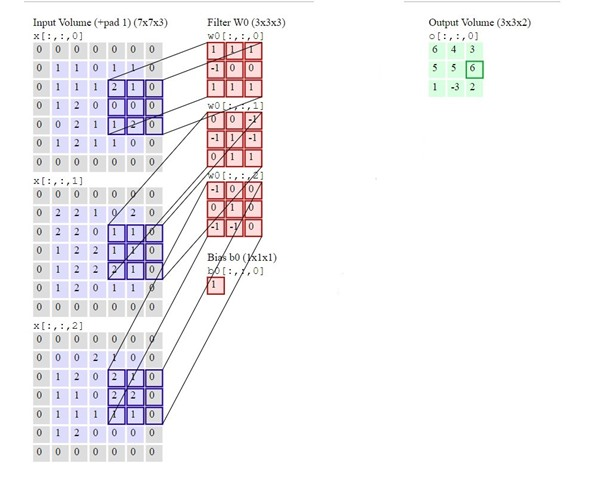This discussion has been locked.
You can no longer post new replies to this discussion. If you have a question you can start a new discussion

# Failed to match cmsis-nn convolution function outputs with hand calculation for IN-channels greater than 1

Hello guys, I am new to ML/AI as a start I am trying to run a convolution layer on M4 by using CMSIS-NN function calls and comparing the output results with a hand calculated outputs for the same inputs and kernel.

I am using a arm_convolve_HWC_q7_basic cmsis-nn function for my convolution layer test and I am successfully able to infer the convolution layer with one channel i.e with cmsis-nn function call and the outputs are exactly matching with my hand calculation outputs.

But the tough part is when i am trying to run the same test with increase in INPUT-channels (greater than one) the outputs of the arm_convolve_HWC_q7_basic function outputs are not matching with my hand calculated outputs.

Please refer to my attached example reference and my code below and help me out with the missing solution thanks in advance.

FYI... I took the same inputs, kernels as shown in the example reference just to make life easy i.e to compare outputs easily.```#include <stdint.h>
#include <stdlib.h>

#include "CMSIS_5/CMSIS/NN/Include/arm_nn_math_types.h"
#include "CMSIS_5/CMSIS/NN/Include/arm_nnfunctions.h"

#define CONV_WT_M4 {1, 1, 1, -1, 0, 0, 1, 1, 1, 0, 0, -1, -1, 1, -1, 0, 1, 1, -1, 0, 0, 0, 1, 0, -1, -1, 0}

const int8_t in_data = {
1, 1, 0, 1, 1, 1, 1, 1, 2, 1, 1, 2, 0, 0, 0, 0, 2, 1, 1, 2, 1, 2, 1, 1, 0, 2, 2, 1, 0, 2, 2, 2, 0, 1, 1, 1, 2, 2, 1, 1, 1, 2, 2, 2, 1, 1, 2, 0, 1, 1, 0, 0, 2, 1, 0, 1, 2, 0, 2, 1, 1, 1, 0, 2, 2, 1, 1, 1, 1, 1, 1, 2, 0, 0, 0};

#define CONV_BIAS_M4 {1}

const int8_t expected_out_data = {
6, 4, 3, 5, 5, 6, 1, -3, 2};

#define CONV_IN_DIM_M4 5
#define CONV_IN_CH_M4 3
#define CONV_KER_DIM_M4 3
#define CONV_STRIDE_M4 2
#define CONV_OUT_CH_M4 1
#define CONV_OUT_DIM_M4 3
#define CONV_BIAS_LSHIFT_M4 0
#define CONV_OUT_RSHIFT_M4 0

static const q7_t conv2_wt[CONV_IN_CH_M4*CONV_KER_DIM_M4*CONV_KER_DIM_M4*CONV_OUT_CH_M4] = CONV_WT_M4;
static const q7_t conv2_bias[CONV_OUT_CH_M4] = CONV_BIAS_M4;
q7_t output_data[CONV_OUT_DIM_M4];
q15_t col_buffer;
q7_t scratch_buffer;

void main(void) {
q7_t* buffer1 = scratch_buffer;

arm_convolve_HWC_q7_basic((q7_t*)in_data, CONV_IN_DIM_M4, CONV_IN_CH_M4, conv2_wt, CONV_OUT_CH_M4, CONV_KER_DIM_M4, CONV_PAD_M4, CONV_STRIDE_M4, conv2_bias, CONV_BIAS_LSHIFT_M4, CONV_OUT_RSHIFT_M4, buffer1, CONV_OUT_DIM_M4, (q15_t*)col_buffer, NULL);

printf("INPUTS\n");
for (int i=0; i< CONV_IN_CH_M4; i++) {
printf("channel %d\n",i);
for (int j=0; j< CONV_IN_DIM_M4; j++) {
for (int k=0; k< CONV_IN_DIM_M4; k++) {
printf("%4d ",in_data[i * CONV_IN_DIM_M4 * CONV_IN_DIM_M4 + j * CONV_IN_DIM_M4 + k]);
}
printf("\n");
}
}
printf("WEIGHTS\n");
for (int i=0; i< CONV_OUT_CH_M4*CONV_IN_CH_M4; i++) {
printf("channel %d\n",i);
for (int j=0; j< CONV_KER_DIM_M4; j++) {
for (int k=0; k< CONV_KER_DIM_M4; k++) {
printf("%4d ",conv2_wt[i * CONV_KER_DIM_M4 * CONV_KER_DIM_M4 + j * CONV_KER_DIM_M4 + k]);
}
printf("\n");
}
}

printf("Expected OUTPUTS\n");
for (int i=0; i< CONV_OUT_CH_M4; i++) {
printf("channel %d\n",i);
for (int j=0; j< CONV_OUT_DIM_M4; j++) {
for (int k=0; k< CONV_OUT_DIM_M4; k++) {
printf("%4d ",expected_out_data[i * CONV_OUT_DIM_M4 * CONV_OUT_DIM_M4 + j * CONV_OUT_DIM_M4 + k]);
}
printf("\n");
}
}
printf("\nM4 OUTPUTS\n");
for (int i=0; i< CONV_OUT_CH_M4; i++) {
printf("channel %d\n",i);
for (int j=0; j< CONV_OUT_DIM_M4; j++) {
for (int k=0; k< CONV_OUT_DIM_M4; k++) {
printf("%4d ",buffer1[i * CONV_OUT_DIM_M4 * CONV_OUT_DIM_M4 + j * CONV_OUT_DIM_M4 + k]);
}
printf("\n");
}
}

if(!memcmp(expected_out_data,buffer1,CONV_OUT_DIM_M4*CONV_OUT_DIM_M4*CONV_OUT_CH_M4))
{
printf("Passed\n");
}
else
{
printf("Failed\n");
}

}```